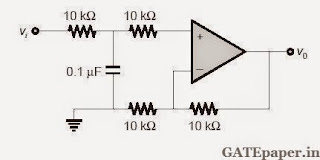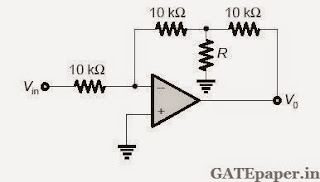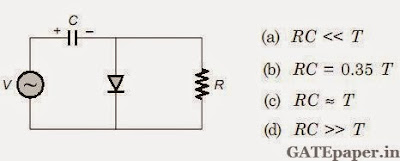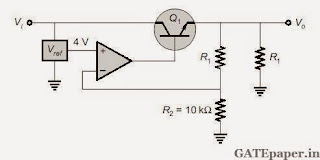### GATE 2015 ECE Analog Circuits - Video Solutions

GATE 2015 ECE – Questions on Analog Circuits with Answers
1. In the circuit shown, assume OP-AMP is ideal then the 3 dB cut off frequency (in Hz) is __________2. Consider the bode plot shown in figure. Assume that all the poles and zeros are real valued. The value of fH – fL (in Hz) is _____________3. At very high frequencies, the peak output voltage Vo (in volts) is ______________4. In the circuit shown, assume that the OP-AMP is ideal. If the gain (Vo/Vin) is –12 , then the value of R (in kΩ) is _________________5. Negative feedback in a closed loop control system DOES NOT
a. Reduce the overall gain
b. Reduce bandwidth
c. Improve disturbance rejection
d. Reduce sensitivity to parameter variation

6. In the circuit shown, assume that the OP-AMP is ideal. The bridge output voltage Vo (in mV) for δ = 0.05 is _______________7. The circuit shown in the figure has an ideal OP-AMP. The oscillation frequency and the condition to sustain the oscillations respectively are ________________8. In the circuit shown, I1 = 80 mA and I2 = 4 mA. Transistors T1 and T2 are identical. Assume that thermal voltage is 26 mV at 27oC. At 50oC, the value of voltage V12 = V1 – V2 (in mV) is _______________9. In the circuit shown, Vo = VOA for switch SW in position A and Vo = VOB for switch SW in position B. Assume that the OP-AMP is ideal. The value of VOB/VOA is ______________10. If the circuit shown has to function as a clamping circuit, then which one of the following conditions should be satisfied for the sinusoidal signal of period T?11. In the bistable circuit shown, the ideal OP-AMP ahs saturation levels of ± 5 volts. The value of R1 (in kΩ) that gives a hysteresis width of 500 mV is _________________12. In the ac equivalent circuit shown, the two BJTs are biased in active region and have identical parameters with β>>1. The open circuit small signal voltage gain is approximately is _________________13. Assuming that the OP-AMP in the circuit shown is ideal, the output voltage Vo (inn volts) is __________14. For the voltage regulator circuit shown,the input voltage (Vin) is 20 V ± 20% and the regulated output voltage (Vout) is 10 volts. Assume the OP-AMP is ideal. For a load RL drawing 200 mA, the maximum power dissipation in Q1 (in Watts) is _________________